# Title 40

## SECTION 1065.645

### 1065.645 Amount of water in an ideal gas.

§ 1065.645 Amount of water in an ideal gas.

This section describes how to determine the amount of water in an ideal gas, which you need for various performance verifications and emission calculations. Use the equation for the vapor pressure of water in paragraph (a) of this section or another appropriate equation and, depending on whether you measure dewpoint or relative humidity, perform one of the calculations in paragraph (b) or (c) of this section. Paragraph (d) of this section provides an equation for determining dewpoint from relative humidity and dry bulb temperature measurements. The equations for the vapor pressure of water as presented in this section are derived from equations in “Saturation Pressure of Water on the New Kelvin Temperature Scale” (Goff, J.A., Transactions American Society of Heating and Air-Conditioning Engineers, Vol. 63, No. 1607, pages 347-354). Note that the equations were originally published to derive vapor pressure in units of atmospheres and have been modified to derive results in units of kPa by converting the last term in each equation.

(a) Vapor pressure of water. Calculate the vapor pressure of water for a given saturation temperature condition, Tsat, as follows, or use good engineering judgment to use a different relationship of the vapor pressure of water to a given saturation temperature condition:

(1) For humidity measurements made at ambient temperatures from (0 to 100) °C, or for humidity measurements made over super-cooled water at ambient temperatures from (−50 to 0) °C, use the following equation: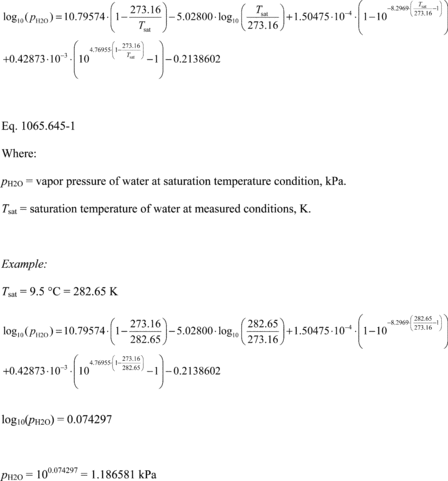(2) For humidity measurements over ice at ambient temperatures from (-100 to 0) °C, use the following equation: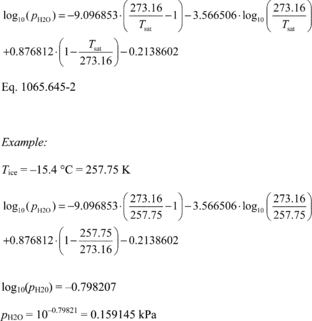(b) Dewpoint. If you measure humidity as a dewpoint, determine the amount of water in an ideal gas, xH20, as follows: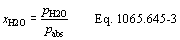Where: xH20 = amount of water in an ideal gas. pH20 = water vapor pressure at the measured dewpoint, Tsat = Tdew. pabs = wet static absolute pressure at the location of your dewpoint measurement. Example: : pabs = 99.980 kPa Tsat = Tdew = 9.5 °C Using Eq. 1065.645-1, pH20 = 1.186581 kPa xH2O = 1.186581/99.980 xH2O = 0.011868 mol/mol

(c) Relative humidity. If you measure humidity as a relative humidity, RH, determine the amount of water in an ideal gas, xH2O, as follows: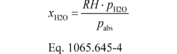Where: xH2O = amount of water in an ideal gas. RH = relative humidity. pH2O = water vapor pressure at 100% relative humidity at the location of your relative humidity measurement, Tsat = Tamb. pabs = wet static absolute pressure at the location of your relative humidity measurement. Example: RH = 50.77% = 0.5077 pabs = 99.980 kPa Tsat = Tamb = 20 °C Using Eq. 1065.645-1, pH2O = 2.3371 kPa xH2O = (0.5077 · 2.3371)/99.980 xH2O = 0.011868 mol/mol

(d) Dewpoint determination from relative humidity and dry bulb temperature. This paragraph (d) describes how to calculate dewpoint temperature from relative humidity, RH. This is based on “ITS-90 Formulations for Vapor Pressure, Frostpoint Temperature, Dewpoint Temperature, and Enhancement Factors in the Range −100 to + 100 °C” (Hardy, B., The Proceedings of the Third International Symposium on Humidity & Moisture, Teddington, London, England, April 1998). Calculate pH20sat as described in paragraph (a) of this section based on setting Tsat equal to Tamb. Calculate pH20scaled by multiplying pH20sat by RH. Calculate the dewpoint, Tdew, from pH20 using the following equation: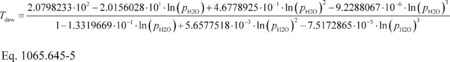Where: ln(pH2O) = the natural log of pH2Oscaled, which is the water vapor pressure scaled to the relative humidity at the location of the relative humidity measurement, Tsat = Tamb Example: RH = 39.61% = 0.3961 Tsat = Tamb = 20.00 °C = 293.15K Using Eq. 1065.645-1, pH2Osat = 2.3371 kPa pH2Oscaled = (0.3961 · 2.3371) = 0.925717 kPa = 925.717 Pa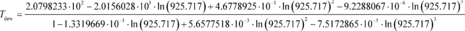[73 FR 37327, June 30, 2008, as amended at 73 FR 59331, Oct. 8, 2008; 75 FR 23048, Apr. 30, 2010; 76 FR 57456, Sept. 15, 2011;79 FR 23796, Apr. 28, 2014; 81 FR 74179, Oct. 25, 2016]